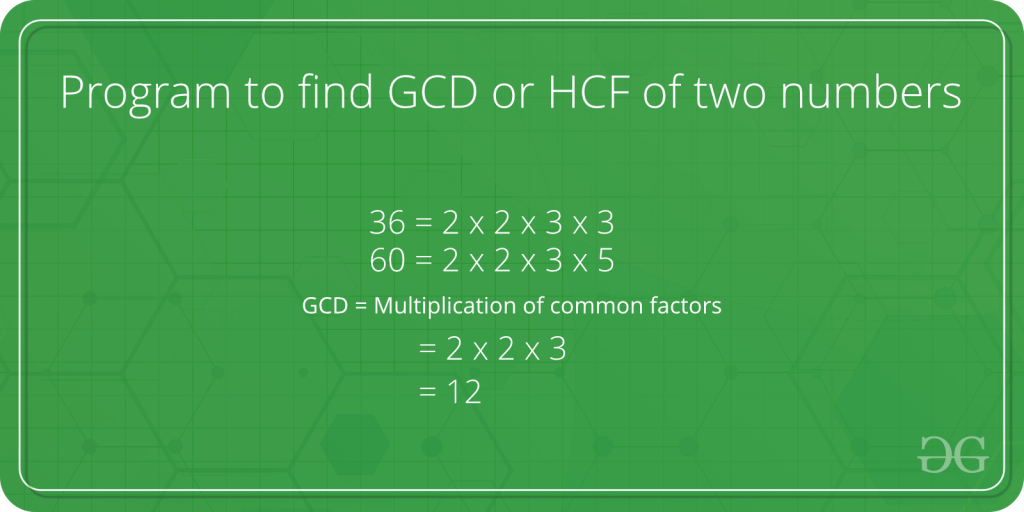Open In App
Related Articles
• Write an Interview Experience
• Java Programs – Java Programming Examples

# Java Program to Find GCD or HCF of Two Numbers

GCD (i.e. Greatest Common Divisor) or HCF (i.e. Highest Common Factor) is the largest number that can divide both the given numbers.Example:

`HCF of 10 and 20 is 10, and HCF of 9 and 21 is 3.`

Therefore, firstly find all the prime factors of both the stated numbers, then find the intersection of all those factors that are present in both the given numbers. And at last, return the product of elements that are in the intersection.

Note: GCD of the two stated numbers doesn’t alter if the smaller number is subtracted from the bigger number.

Example 1:

## Java

 `// Java program to find GCD of two numbers``class` `GFG {``    ``// Gcd of x and y using recursive function``    ``static` `int` `GCD(``int` `x, ``int` `y)``    ``{``        ``// Everything is divisible by 0``        ``if` `(x == ``0``)``            ``return` `y;``        ``if` `(y == ``0``)``            ``return` `x;`` ` `        ``// Both the numbers are equal``        ``if` `(x == y)``            ``return` `x;`` ` `        ``// x is greater``        ``if` `(x > y)``            ``return` `GCD(x - y, y);``        ``return` `GCD(x, y - x);``    ``}`` ` `    ``// The Driver method``    ``public` `static` `void` `main(String[] args)``    ``{``        ``int` `x = ``100``, y = ``88``;``        ``System.out.println(``"GCD of "` `+ x + ``" and "` `+ y``                           ``+ ``" is "` `+ GCD(x, y));``    ``}``}`

Output

```GCD of 100 and 88 is 4
```

Similarly, you can find the GCD or HCF of any two given numbers.

An efficient solution is to utilize a modulo operator in the Euclidean algorithm which is the foremost algorithm applied for this topic.

Example 2:

## Java

 `// Java program to find GCD of two ``// numbers using Euclidean algorithm``class` `geeksforgeeks {``    ``// Function to return gcd of x and y``    ``// recursively``    ``static` `int` `GCD(``int` `x, ``int` `y)``    ``{``        ``if` `(y == ``0``)``            ``return` `x;``        ``return` `GCD(y, x % y);``    ``}`` ` `    ``// The Driver code``    ``public` `static` `void` `main(String[] args)``    ``{``        ``int` `x = ``47``, y = ``91``;``        ``System.out.println(``"The GCD of "` `+ x + ``" and "` `+ y``                           ``+ ``" is: "` `+ GCD(x, y));``    ``}``}`

Output

```The GCD of 47 and 91 is: 1
```